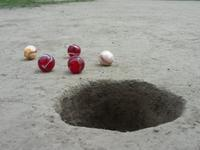# Beginning 2879

Juro, Fero, and Mišo played bullets. In the beginning, everyone had the same number. In the first game, Juro won 4 bullets, and Fero 5 (that means Mišo lost nine bullets). In the second game, Mišo won 7 balls, and Fero lost four balls. In the third game, Mišo lost three bullets, and Juro lost two bullets.
Find out if he had more Juro or Mišo bullets at the end. How much more?

d =  4
f =  6
j =  -1
m =  -5

### Step-by-step explanation:

j = +4-3-2
f = +5-4+5
m = -9+7-3
d = j-m

j = -1
f = 6
m = -5
d-j+m = 0

Pivot: Row 1 ↔ Row 4
d-j+m = 0
f = 6
m = -5
j = -1

Pivot: Row 3 ↔ Row 4
d-j+m = 0
f = 6
j = -1
m = -5

m = -5/1 = -5
j = -1/1 = -1
f = 6/1 = 6
d = 0+j-m = 0-1+5 = 4

d = 4
f = 6
j = -1
m = -5

Our linear equations calculator calculates it.Did you find an error or inaccuracy? Feel free to write us. Thank you!